#### You may also like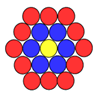### Counting Counters

Take a counter and surround it by a ring of other counters that MUST touch two others. How many are needed?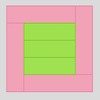### Cuisenaire Squares

These squares have been made from Cuisenaire rods. Can you describe the pattern? What would the next square look like?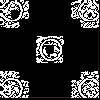### Doplication

We can arrange dots in a similar way to the 5 on a dice and they usually sit quite well into a rectangular shape. How many altogether in this 3 by 5? What happens for other sizes?

# Counting Stick Conjectures

##### Age 5 to 11Challenge Level

Have you ever seen a counting stick? You might have one in your classroom at school. Here is a photo of one: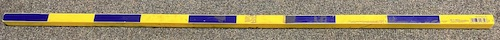What do you see?
What do you notice?
What would you like to ask?

Here is a simple picture of the counting stick: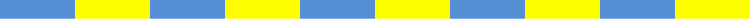How many rectangles can you see? (The rectangles may be different sizes.)

Once you've had a think about how many rectangles there might be, click below to see what Zoya thought.

Zoya says:

"I can see some small rectangles, which are either yellow or blue.
I can also see some bigger rectangles, made of two of the small ones. And some that are even bigger too.
I tried to count all the rectangles but I got very confused."

What might you suggest that would help Zoya?

Again, have a think and then click below to see what Max suggested.

Max says:

"Perhaps it would be easier if we started with a smaller counting stick.
We could try a counting stick with just two of the smallest rectangles, like this: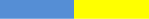I think that has two small rectangles and one larger one, which makes three rectangles altogether.

Then we could try a counting stick with three smallest rectangles, like this: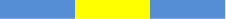This time I can see three very small rectangles, two blue and one yellow.

I can also see a rectangle which is made up of two of these small ones, which I've outlined in red: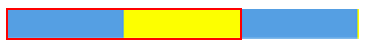And there's another one like this: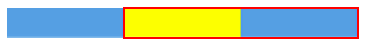And then there is one large rectangle: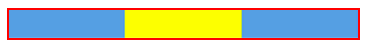So, I think that makes six altogether.

If we do this a few more times with different sized sticks, perhaps we'll see a pattern."

Can you see how Max worked out the total number of rectangles each time?

Try out his suggestion. How many rectangles are there on a counting stick which is made up of four small squares? Five squares? Six...?

What do you notice? Can you see a pattern?
Is there a quick way to work out how many rectangles there would be, for a counting stick with 100 sections? Or 1000? Or...

How can you be sure that what you have noticed will always be true?

Mathematicians aren't usually satisfied with a few examples to convince themselves that something is always true.
Can you provide an argument that would convince mathematicians?